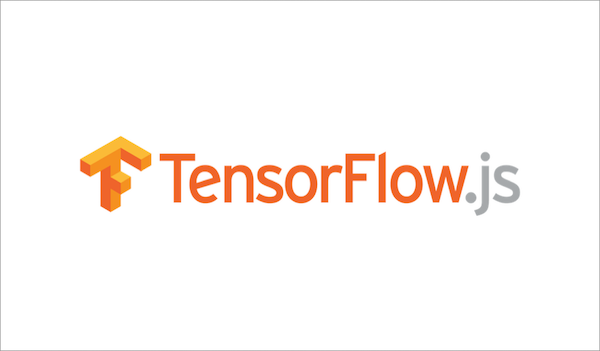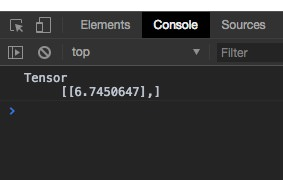# 從零開始的機器學習生活 - Parcel.js 與 TensorFlow.js### Parcel.js 快速打包工具

Parcel.js 是一套快速的打包工具，至於有多快速看看他的官方簡介就知道了安裝也只要 `npm install -g parcel-bundler` 詳細可以觀看 快速开始 有了 Parcel.js 可以讓我們很方便的開發單一頁面的網站如果要開發大型網站還是使用其他工具會比較好。

### TensorFlow.js

`yarn init -y` 或是 `npm init -y`

`yarn add @tensorflow/tfjs`
`npm install @tensorflow/tfjs`

``````import * as tf from '@tensorflow/tfjs';

// Define a model for linear regression.
const model = tf.sequential();

// Prepare the model for training: Specify the loss and the optimizer.
model.compile({loss: 'meanSquaredError', optimizer: 'sgd'});

// Generate some synthetic data for training.
const xs = tf.tensor2d([1, 2, 3, 4], [4, 1]);
const ys = tf.tensor2d([1, 3, 5, 7], [4, 1]);

// Train the model using the data.
model.fit(xs, ys, {epochs: 10}).then(() => {
// Use the model to do inference on a data point the model hasn't seen before:
model.predict(tf.tensor2d(, [1, 1])).print();
});
``````